# HOW TO SOLVE PROBLEMS ON COMPOUND INTEREST-LEARN SERIES

Hi Bankersdaily Aspirants,

Aspirants as you all know IBPS RRB exams are heading soon ,we had started our Preparation & we had Started Learn Series for Neophyte’s in which we are discussing on the each type of problem in all the topics in Aptitude,Reasoning and English Section.

## INTRODUCTION TO COMPOUND INTEREST:

Compound interest is the addition of interest to the principal sum of a loan or deposit, or in other words, interest on interest.

In IBPS Exam,2 Questions are asked from this section.

### FORMULAS FOR COMPOUND INTEREST:

1. Let Principal = P, Rate = R% per annum, Time = n

####When interest is compounded Half-yearly: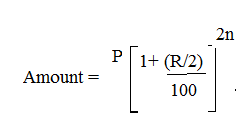#### When interest is compounded Quarterly: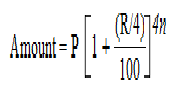#### When interest is compounded Annually but time is in fraction, say 3 2/5 years: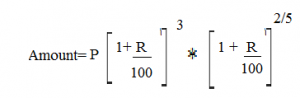#### When Rates are different for different years, say R1%, R2%, R3% for 1st, 2ndand 3rd year respectively.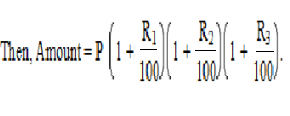#### Present Worth of Rs.x due n years hence is given by: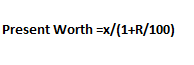### COMPOUND INTEREST:

 PRINCIPAL CI IN YEARS YEARS TOTAL CI AMOUNT 1000 100 1ST YEAR 100 1100 1000 110 2ND YEAR 210 1210 1000 121 3RD YEAR 331 1331

### SIMPLE INTEREST:

 PRINCIPAL SI IN YEARS YEARS TOTAL SI AMOUNT 1000 100 1ST YEAR 100 1100 1000 100 2ND YEAR 200 1200 1000 100 3RD YEAR 300 1300

#### NOTE:

1.For 1st year SI=CI

2.Variation of Interest Starts from 2nd Year.

3.In CI interest and Amount in 2consecutive years also increases by r%

#### TYPE 1:

1.If a man invests Rs.10000 at the rate of 20% per annum for 2 1/2 years.What is the amount of the man if his principal is compounded annually?

Explanation

Amount=10000(1+20/100)²*(1+20/100*1/2)¹

=10000[(120/100)*(120/100)]*(110/100)

=15840

### DIFFERENT RATES FOR DIFFERENT YEARS:

1.If a man invests principal of Rs.10000, with different rate of interest that is for first 2 years Rate of interest is 10% for the next year Rate of Interest is 20% and then for next 1 year is 25% per annum which is compounded annually, What will be the amount for 4years?

Explanation

Amount=P{(1+r1/100)t1  * (1+r2/100)t2 * (1+r3/100)t3}

=10000(1+10/100)² *(1+20/100)¹ * (1+25/100)¹

=10000*( 110/100)*(110/100) *(120/100) *(125/100)

=Rs.18150

### WHEN INTEREST IS COMPOUNDED ANUALLY, HALF YEARLY, QUARTERLY:

#### 1.A man invests principal of Rs.10000 at the rate of 20%per annum with interest compounded half yearly for 2 years.What is the Amount he will have at the end of 2years?

Explanation

P=Rs.10000

R=20%per annum

Interest compounded half yearly for 2 years

A=10000(1+(20/100*1/2))²*²

=10000*(110/100) *(110/100) *(110/100) *(110/100)

Amount=Rs.14641

### BASED ON EFFICIENCY:

1.A sum amounts to twice in 3 years  at Compound Interest.In how many years it becomes 8 times at same rate r%?

Explanation

Given Amount=2P in 3 years

Amount=P(1+r/100)n

2P =P(1+r/100)ᶾ

2= (1+r/100)ᶾ

To become 8 times 2 should be cubed

2ᶾ= (1+r/100)3*3

8=(1+r/100)9

It becomes 8 times in 9 years

ALTERNATE METHOD:

M times=t1 years

N times=t2 years

m⅓ =n¹/t2

2⅓=8¹/t2

2⅓ =2ᶾ*

Base are equal

1/3=3*1/t2

t2=9

9 years are required for a sum to become 8 times

=PR²/100²

### FOR 3 YEARS:

=PR²(300+R)/100³

1.The difference between Simple interest and Compound Interest in(Rs.) on Rs.1200 for one year at 10% per annum reckoned  half yearly is?

Explanation

Since the interest is compounded half yearly  therefore rate=10/2 =5%

CI-SI=PR²/100²

=1200*5*5/1000

=30000/10000

### =Rs.3

2.If the difference between CI and SI on a certain sum of money for 3 years at 10% per annum is Rs.93.Find the sum(in Rs.)

Explanation

Sum= Difference*100³/r³(300+r)

=93*100*100*100/(10*10*310)

=Rs.3000